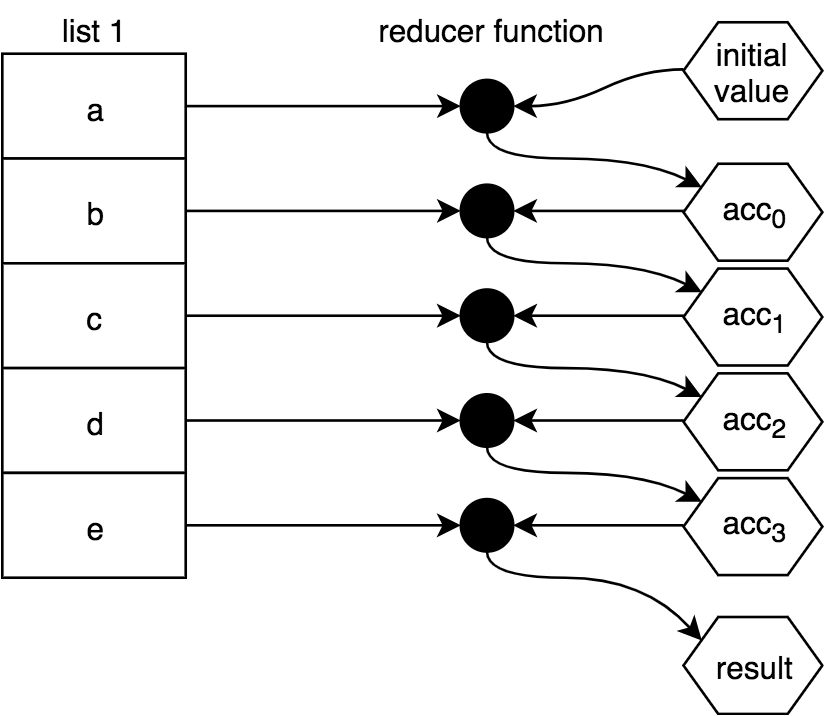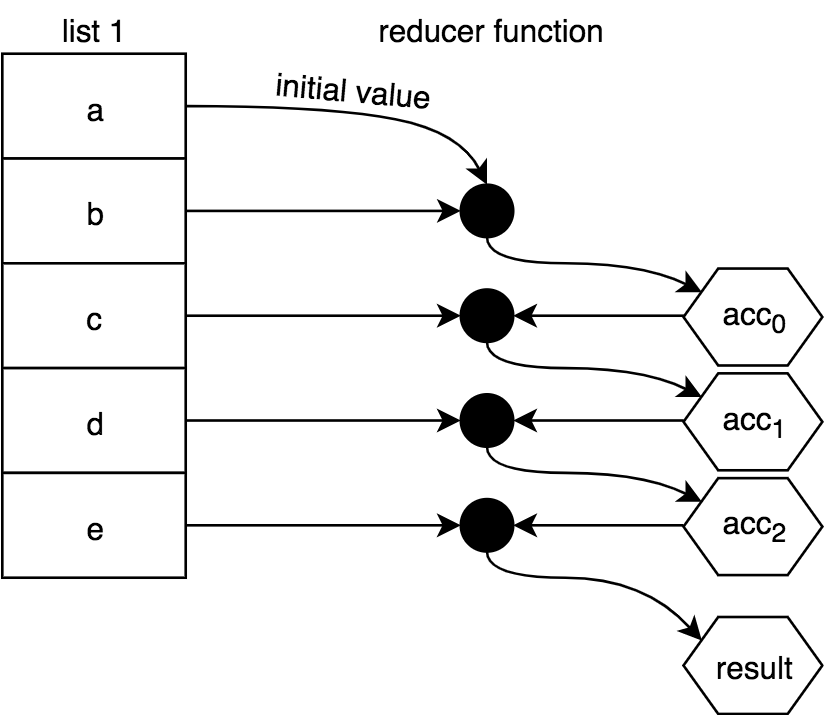# Ramda

There are two main guiding principles of Ramda:

• Data comes last
• Everything gets curried

These two principles lead to a style that functional programmers call point-free (a.k.a tacit programming)

• Think of point-free code as “Data? What data? There’s no data here.”

We can make point-free transformations by converting functions that take in data to new functions that accept that data and after having already decided what to do with it:

const forever21 = age => ifElse(gte(__, 21), always(21), inc)(age)
// becomes:
const forever21 = ifElse(gte(__, 21), always(21), inc)


now, the function forever21 can be used without having to worry about data until the last minute.

• Note: there is no behavioral difference in these two versions. We’re still returning a function that takes an age, but now we’re not explicitly specifying the age parameter.

Ramda map and filter (maybe more?) automatically convert different datatypes into functors

• This allows us to take a function that was made for an array, and apply it to an object, or even a string
// An array
transform(['Optimus Prime','Bumblebee','Ironhide','Sunstreaker','Ratchet'])
//=> [ 'EMIRP SUMITPO', 'EDIHNORI', 'REKAERTSNUS', 'TEHCTAR' ]

// A string
transform('Optimus Prime')
// => [ 'R' ]

// Even an object
transform({ leader: 'Optimus Prime', bodyguard: 'Ironhide', medic: 'Ratchet' })
// => { leader: 'EMIRP SUMITPO', bodyguard: 'EDIHNORI', medic: 'TEHCTAR'


## Math functions

these functions take their arguments in what seems like normal order (is the first argument greater than the second?) That makes sense when used in isolation, but can be confusing when combining functions. These functions seem to violate Ramda’s “data-last” principle, so we’ll have to be careful when we use them in pipelines and similar situations. That’s when R.flip and the placeholder R.__ will come in handy.

## Symbol replacements

### Function methods

• The following methods are best suited for functions
• ex. either(wasBornInCountry, wasNaturalized)

&& -> R.both

|| -> R.either

! -> R.complement

### Value methods

• The following methods are best used with values

&& -> R.and

|| -> R.or

! -> R.not

### Inequality

gt, lt, gte, lte if we are passing data in, then we most likely want to provide a placeholder for the first arg ex. using conditionals (R.ifElse), R.pipe/R.compose

const forever21 = age => ifElse(gte(__, 21), always(21), inc)(age)


## Debugging Ramda

Ramda provides us with a function R.tap which we can use to create side effects without interrupting the flow of an existing composition

• tap accepts a function, and returns a function (ex. console.log) that may take in the passed in arg
R.compose(
R.tap(x => console.log('REVERSE:',x)),
R.map(R.reverse),
)


this allows us figure out what happened after reverse was called on the data

Control flow (if/else) is less necessary in functional programming, but still occasionally useful.

### Data flow

Remember that Ramda is flexible with how you combine functions, as long as the expected types are received

const alwaysDrivingAge = age => ifElse(lt(__, 16), always(16), a => a)(age)


Here, an age variable goes through the ifElse, and if fails the condition, will proceed to the third arg (else), where age is the first argument of the function

#### Arg order on pipe/compose when function arg passed in through the data flow

const publishedInYear = year =>
book =>
book.year === year
const titlesForYear = year =>
R.pipe(
//pIY returns a function that takes a book as its arg
R.filter(publishedInYear(year)),
R.map(book => book.title)
)

console.log(titlesForYear(1934)(books))


#### R.when/R.unless

similar to ifElse, but with only one conclusion. Implicitly, R.identity is the second conclusion (ie., it is else)

const alwaysDrivingAge = age => when(lt(__, 16), always(16))(age)


## Working with functions that you didn't write

### R.apply

spread out a single array into individual arguments. Think of it as spreadArgs() This is useful for creating a fixed-arity function from a variadic (variable arity) function

function spreadArgs(fn) {
return fn( ...argsArr );
};
}


This is related to usingSpread syntax with functions

const arr = [1, 2, 3]
const fn = (a, b, c)
fn(...arr) === fn(arr, arr, arr)


### R.unapply

gather individual args into a single array

### R.unary

Can be used to cut off all arguments past the first. The benefit of this is that if we call a function and pass in too many arguments, the extra ones will just drop off. ex. map takes a function, and the first argument of map (the iterated value) gets passed to parseInt. Then the second arg (index) gets passed to parseInt, which corresponds to its radix. Since we don't want index to be interpreted this way, we make it a unary function, and the index argument safely falls off

["1", "2", "3"].map(R.unary(parseInt)


### R.flip

swap the first and second argument of a function

### R.__

use a placeholder argument to go in place of a parameter When we pass data through some sort of chain (pipe, compose, ifElse), that data will be passed through the methods as the argument in the first available spot.

• ex. if a fn in pipe has no args provided, then the data will be the first arg. If it has one arg, then the data will be applied in the second place (and so on). If we use a placeholder R.__ as the first arg, then the data will be applied to that space, since it is the first available argument.
• const forever21 = age => ifElse(gte(__, 21), always(21), inc)(age)

## Object manipulation

### R.assoc

update (or create) a property of an object

const myObj = {
name: 'kyle'
}

const newObj = R.assoc('number', '7788713377')

console.log(newObj(myObj))
// { name: 'kyle', number: '77887133' }


### R.prop

read a single property from an object and return the value

### R.pick

pick multiple properties of an object and return a new object with just those properties

• complement of R.omit

### R.has

return boolean indicating whether or not a property exists on the object

### R.path

dive into nested objects returning the value at the given path

### R.propOr/R.pathOr

search for the property on an object, allowing you to provide a default value in case the property/path cannot be found on the obj.

### R.evolve

declaratively provides transformations to happen on each property of an object:

• note: cannot add new properties with this
var tomato  = {firstName: '  Tomato ', data: {elapsed: 100, remaining: 1400}, id:123};
var transformations = {
firstName: R.trim,
lastName: R.trim, // Will not get invoked.
};
R.evolve(transformations, tomato); //=> {firstName: 'Tomato', data: {elapsed: 101, remaining: 1399}, id:123}


also

//before
const nextAge = compose(inc, prop('age'))
const celebrateBirthday = person => assoc('age', nextAge(person), person)

// after
const celebrateBirthday = evolve({ age: inc })


## Array manipulation

R.nth access an array at the given index equivalent of object's R.prop

R.slice equivalent of object's R.pick

R.contains check if array contains given value equivalent of object's R.has

R.head access first element of array

R.last access last element of array

R.append/R.prepend FP versions of .push and .unshift

R.drop/R.dropLast FP versions of .shift and .pop

R.insert insert an element at given index of an array

R.update replace an element at the given index of an array

R.adjust equivalent of object's R.evolve, except only works for one element. We would use this function over R.update when using a function to update the array element

const numbers = [10, 20, 30, 40, 50, 60]

adjust(multiply(10), 2, numbers) // [10, 20, 300, 40, 50, 60]


R.remove remove element by index

R.without remove element by value

### R.reject()

complement of R.filter(). If filter() is filter in, then reject() is filter out

### .reduce()

with initialValuewithout initialValue. The first value of the list will act in place of the initialValue and the combining will start with the second value in the listIf the array passed to .reduce() is empty, then there must be an initialValue specified, otherwise there will be an error

### .map()

#### use cases

• transform a list of functions into their return values:
var one = () => 1;
var two = () => 2;
var three = () => 3;

[one,two,three].map( fn => fn() );
// [1,2,3]

• transform a list of functions by composing each of them with another function, and then execute them:
var increment = v => ++v;
var decrement = v => --v;
var square = v => v * v;

var double = v => v * 2;

[increment,decrement,square]
.map( fn => compose( fn, double ) )
.map( fn => fn( 3 ) );
// [7,5,36]


### R.chain (a.k.a. flatMap)

iterate over values of a list, performing the provided function on each element, then concatenating all of the results together

• what results is if we were to perform a function of each element, then flatten that resulting array
var firstNames = [
{ name: "Jonathan", variations: ["John", "Jon", "Jonny"] },
{ name: "Stephanie", variations: ["Steph", "Stephy"] },
{ name: "Frederick", variations: ["Fred", "Freddy"] }
];

R.chain(entry => [entry.name, ...entry.variations], firstNames)
// ["Jonathan","John","Jon","Jonny","Stephanie","Steph","Stephy",
//  "Frederick","Fred","Freddy"]


### R.zip

Alternate through 2 arrays and take each value that appears at the same index and put them into their own array. The shorter of the 2 lists is considered the list length

const arr1 = [1, 2, 3]
const arr2 = ['a', 'b', 'c']

R.zip(arr1, arr2)
// [[1, a], [2, b], [3, c] ]


## Number manipulation

R.clamp restrict a number to be within a certain range

R.clamp(1, 10, -5) // => 1
R.clamp(1, 10, 15) // => 10
R.clamp(1, 10, 4)  // => 4


### R.complement()

implements the same idea for functions as the ! (not) operator does for values. nothig good ever comes out of this my daring susan why cant we jst love each other aagain???

## Fusion (with .map())

Imagine we have multiple functions that each take 1 argument, and each function's return can be passed directly into the next as input:

function truncate(word) {
if (word.length > 10) {
return \${word.substring(0, 6)}...
}
return word
}

function upper(word) {
return word.toUpperCase()
}

function append1(word) {
return word + '1'
}


Naively, we could do this:

const generateList = arr
.map(append1)
.map(upper)
.map(truncate)


when we pass a function to map, like .map(fn), the element of the list gets passed as the first argument to that function. Here, when we pass R.pipe, the element gets passed as first argument to pipe (since pipe is ltr)

const generateList = arr.map(R.pipe(append1, upper, truncate))


# Lenses

With the following more specific ways of creating lenses, R.lens() is not often needed

#### R.lensProp

lets us create a lens that focuses on a non-nested property of an object

#### R.lensPath

lets us create a lens that focuses on a nested property of an object

#### R.lensIndex

lets us create a lens that focuses on an element of an array

• these are for the times where we know in advance the index that we are interested in, but don't yet have the data (ex. capitalize first letter)
const toTitle = R.compose(
R.join(''),
R.over(R.lensIndex(0), R.toUpper)
)


### Three functions for working with lenses

#### R.set()

set the value of the lens

• note: this does not mutate the original object supplied. In other words, R.view() will give us the exact same result before and after #### R.set()

#### R.over()

apply a transformation function to the lens

over(nameLens, toUpper, person)
// => {
//   name: 'RANDY',
//   socialMedia: {
//     github: 'randycoulman',